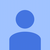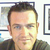0

# How to perform mouse click hit testing on ModelVisual3d models in Helixtoolkit

Suran Mahawithana 7 years ago updated by Joe Soap 3 years ago

I am developing an application using Helix toolkit in which users are given control to click on the individual 3d models that have loaded and manipulate them independantly. 3d model loader in my application can load ModelVisual3D type models. the tutorials so far I could find were on performing hit testing on Model3D type models which didn't work with ModelVisual3D type whatsoever. Can anybody help ?Suran,

Not sure if this helps exactly, but I recently did this with a WPF project consisting of a window, a grid, and a HelixViewport3D object. Here's the XAML and the MouseDown event code

<code>

private void vp_raw_MouseDown(object sender, System.Windows.Input.MouseButtonEventArgs e)

{
System.Windows.Point mousept = e.GetPosition(vp_raw);
Debug.Print("At top of MouseDown event, Mouse position " + mousept.ToString());

//code to print out current selected point list
if (selPointsVisual != null)
{
int selcount = selPointsVisual.Points.Count;
for (int i = 0; i < selcount; i++)
{
Point3D selpt = selPointsVisual.Points[i];
Debug.Print("selected pt at index " + i + " = ("
+ selpt.X.ToString("F2") + ", "
+ selpt.Y.ToString("F2") + ", "
+ selpt.Z.ToString("F2") + ")");
}
}
else
{
Debug.Print("No selected points exist");
}

//Step1: Get the current transformation matrix
//get a reference to the visual element containing datapoints
int chldcount = vp_raw.Children.Count;
PointsVisual3D p3dvis = null; //temp object
for (int i = 0; i < vp_raw.Children.Count; i++)
{
Visual3D Vis = (Visual3D)vp_raw.Children[i];
string visnamestr = Vis.GetName();
Debug.Print("visual element [" + i + "]'s name is " + visnamestr);
if (visnamestr != null && visnamestr.Contains("magpoints"))
{
p3dvis = (PointsVisual3D)Vis;
break;
}
}

//use the temp object to get the transformation matrix for the parent viewport
Matrix3D m3D = p3dvis.GetViewportTransform();

//Debug.Print(m3D.ToString());
//Point3D xfrmpt = m3D.Transform(new Point3D(0,0,0));
//string ctrptstr = xfrmpt.X.ToString("F2") + ", "
// + xfrmpt.Y.ToString("F2") + ", "
// + xfrmpt.Z.ToString("F2");
//Debug.Print("(0,0,0) transforms to " + ctrptstr);

//Step2: transfer any currently selected points back to main points list unless SHIFT key is down
if ((Keyboard.Modifiers & ModifierKeys.Shift) != ModifierKeys.Shift)
{
if (selPointsVisual != null)
{
int selcount = selPointsVisual.Points.Count;
int magptcount = m_pointsVisual.Points.Count;
Debug.Print("selcount = " + selcount + ", magptcount = " + magptcount);
if (selcount > 0)
{
for (int i = 0; i < selcount; i++)
{
//get selected point
Point3D selpt = selPointsVisual.Points[i];
string selptstr = selpt.X.ToString("F2") + ","
+ selpt.Y.ToString("F2") + ","
+ selpt.Z.ToString("F2");

Debug.Print("Moving selected pt (" + selptstr + ") to pointsVisual. Before add, pt count is "
+ m_pointsVisual.Points.Count);

int newcount = m_pointsVisual.Points.Count;

//check that point got added properly
Point3D newpt = m_pointsVisual.Points[newcount - 1];
string newptstr = newpt.X.ToString("F2") + ","
+ newpt.Y.ToString("F2") + ","
+ newpt.Z.ToString("F2");

Debug.Print("point (" + newptstr + ") added to pointsVsiual at index " + (newcount - 1)
+ ". Count now " + newcount);
}
selPointsVisual.Points.Clear();
}
}
}

//Step3: copy pointsVisual.Points index of any selected points to m_selidxlist
m_selidxlist.Clear();
int ptidx = 0;
foreach (Point3D vispt in m_pointsVisual.Points)
{
Point3D xpt = m3D.Transform(vispt);
double distsq = (mousept.X - xpt.X) * (mousept.X - xpt.X) + (mousept.Y - xpt.Y) * (mousept.Y - xpt.Y);
double dist = Math.Sqrt(distsq);
if (dist < 5)
{
m_selidxlist.Add(ptidx); //save the index of the point to be removed

string visptstr = vispt.X.ToString("F2") + ","
+ vispt.Y.ToString("F2") + ","
+ vispt.Z.ToString("F2");

string xfrmptstr = xpt.X.ToString("F2") + ", "
+ xpt.Y.ToString("F2") + ", "
+ xpt.Z.ToString("F2");
Debug.Print("dist = " + dist.ToString("F2") + ": mousepoint " + mousept.ToString()
+ " matches with magpt[" + ptidx + "] ( " + visptstr + "), "
+ "which transforms to " + xfrmptstr);
}

ptidx++;
}

//move selected points from pointsVisual3D to selpointsVisual3D
int selidxcount = m_selidxlist.Count;
Debug.Print("Selected Index List Contains " + selidxcount + " items");

if (selidxcount > 0)
{

int newcount = 1;
//06/06/16 new try at moving selected points from m_pointsVisual to selPointsVisual
for (int selidx = selidxcount - 1; selidx >= 0; selidx--)//have to work from top down to avoid crashes
{
//retrieve selected point from pointsVisual collection
int visptidx = m_selidxlist[selidx]; //this is the index into pointsVisual.Points collection
Point3D selvispt = new Point3D();
selvispt.X = m_pointsVisual.Points[visptidx].X;
selvispt.Y = m_pointsVisual.Points[visptidx].Y;
selvispt.Z = m_pointsVisual.Points[visptidx].Z;
string selvisptstr = selvispt.X.ToString("F2") + ","
+ selvispt.Y.ToString("F2") + ","
+ selvispt.Z.ToString("F2");
Debug.Print("selected pt (" + selvisptstr + ") added to m_selpoints Collection. Count now "
+ newcount);

//testing - print out added point

//remove this point from m_pointsVisual collection
Debug.Print("removing point at m_pointsVisual[" + m_selidxlist[selidx] + "]");
m_pointsVisual.Points.RemoveAt(m_selidxlist[selidx]);
}

//DEBUG!!
Debug.Print("\r\n selPointsVisual Points collection contains the following points");
foreach (Point3D pt3 in selPointsVisual.Points)
{
string newptstr3 = pt3.X.ToString("F2") + ","
+ pt3.Y.ToString("F2") + ","
+ pt3.Z.ToString("F2");
Debug.Print("Selected pt (" + newptstr3 + ")");
}
}
vp_raw.UpdateLayout();//refresh the 'raw' view
}

</code>

And the XAML

<code>

<Window

xmlns="http://schemas.microsoft.com/winfx/2006/xaml/presentation"
xmlns:x="http://schemas.microsoft.com/winfx/2006/xaml"
xmlns:d="http://schemas.microsoft.com/expression/blend/2008"
xmlns:mc="http://schemas.openxmlformats.org/markup-compatibility/2006"
xmlns:local="clr-namespace:MyWPFMagViewer2"
xmlns:h="http://helix-toolkit.org/wpf" x:Class="MyWPFMagViewer2.MainWindow"
mc:Ignorable="d"
Title="Raw Magnetometer Data" Height="350" Width="525">
<Grid>

<h:HelixViewport3D x:Name="vp_raw" Margin="0" MouseDown="vp_raw_MouseDown" CoordinateSystemHorizontalPosition="Center" CoordinateSystemVerticalPosition="Center" Orthographic="True" ShowCoordinateSystem="True" Title="Title Goes Here" Grid.ColumnSpan="2"/>

</Grid>
</Window>

</code>

Sorry, no preview feature, so cant tell how this is going to look :-(

FrankAmazing! With a little bit of tweaking here and there, it worked, practically out the box. Thanks Frank. Everybody make sure to Thank Frank.